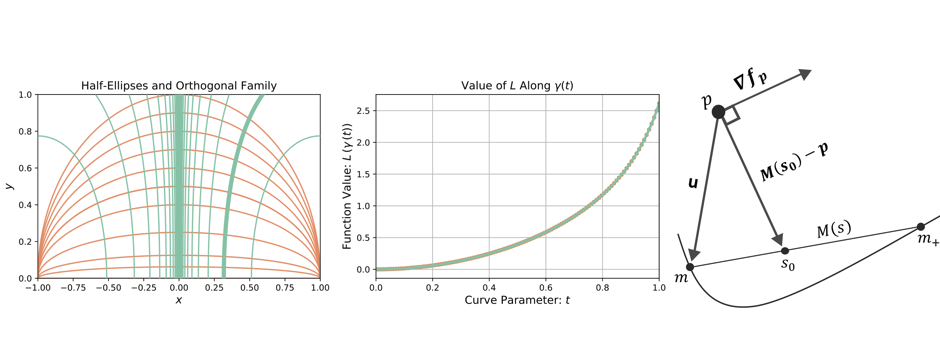# Active Manifolds: Reducing high-dimensional data to 1-DWhen fitting the parameters of a scientific model, it may be the case that real data is expensive or difficult to acquire. A natural question then arises – how can one get the most accurate understanding of a physical situation when given sparse or limited data? Active Manifolds (AM) is a technique for reducing the dimension of regression problems when the function in question involves a high number of input dimensions relative to the data acquired. In this fashion, Active Manifolds extends the Active Subspace methods of Paul Constantine, which use techniques from principal component analysis (PCA) applied to the gradient of a model function in order to compute a lower-dimensional affine subspace where the unknown function changes most on average. Similarly, AM seeks to find and exploit a 1-D submanifold on which the function exhibits the most change.

More precisely, given samples of an unknown function $f:\mathbb{R}^m \to \mathbb{R}$ and its gradient $\nabla f$, our method computes an integral curve of $\nabla f$ (an active manifold) inside the function domain. This provides a 1-D object that encompasses almost all of the change in $f$, and which can be used to (formally) construct the quotient space $\mathbb{R}^m/{\sim}$ (isomorphic to $\mathbb{R}$) where each point $x_0 \in \mathbb{R}^m$ is identified with its level set submanifold $\{ y \in \mathbb{R}^m \vert \, f(y) = f(x_0) \}$. The utility of this is that, with a suitable approximation of the quotient map $\pi: \mathbb{R}^m \to \mathbb{R}^m /{\sim}$, the arbitrarily large regression problem in question becomes essentially one-dimensional; recovering $f$ along the active manifold is analogous to recovering the factorization map $\hat f: \mathbb{R}^m/{\sim} \to \mathbb{R}$ which completely characterizes the range of $f$. We provide a mathematical justification for this method, a basic algorithm for running the program, and a comparison against previous results involving magnetohydrodynamic simulations which were obtained using Active Subspaces.

The benefits of the AM method include increased accuracy (at more computational cost), greatly improved visualization capabilities, more refined sensitivity analysis, and complete reduction to a 1-D problem. On the other hand, very little is known about the algorithmic accuracy or dependence on data, so further work in this area should include obtaining rigorous estimates on these quantities, as well as investigating method sensitivity with respect to the amount/spread of data, and bounding the complexity and convergence of the involved algorithms.

# Relevant Publications

Robert A. Bridges, Anthony D. Gruber, Christopher Felder, Miki Verma, Chelsey Hoff. Active Manifolds: A non-linear analogue to Active Subspaces, Volume 97: International Conference on Machine Learning (2019). Preprint version available here.

See also the code page on this website.